# B-树和B+树¶

## 二、定义¶

$$B$$ 树（又称 $$B-$$ 树）和 $$B+$$ 树其实差别不是很大，所以我会着重介绍 $$B$$ 树，然后最后指出 $$B+$$ 树的不同点

$$B$$ 树，又称 多路平衡查找树$$B$$ 树中 所有结点的孩子个数的最大值 称为 $$B$$ 树的 ，通常用 $$m$$ 表示。

• ①若根节点不是 叶结点 ，则至少有两个子树（即一个关键字）
• ②除了根结点外，其他每个结点至少有 $$\frac{m}{2}$$ 个子树，最多有 $$m$$ 个子树，对应的每一个结点至少有 $$\left \lceil \frac{m}{2} -1 \right \rceil$$ 个关键字
• ③每一个结点的关键字从左到右升序排列
• $$B$$ 树是一个严格的多路平衡查找树，它的左右子树的高度室相等的，且叶结点处于同一层（即所有结点的平衡因子都为 $$0$$

PS：这里需要注意一下，在王道的书上是将叶结点（P285页）定义成了不存在的一层，但是在严蔚敏的书上定义的就是最后一层有关键字的结点，比如下图中，王道将第四层定义为叶子节点，严蔚敏的书上认为叶子结点（终端节点）是第三层，而笔者也认为第三层才是叶子节点或者称为终端节点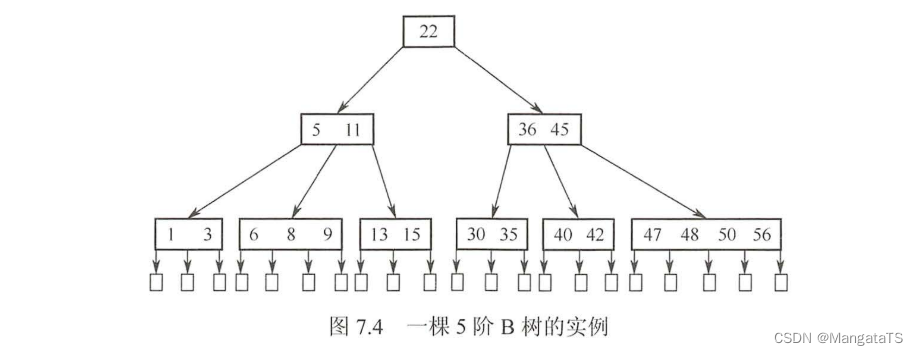## 三、原理¶

### 3.1 树的高度¶

• 至少高度： 既然是至少高度，那么我们应该尽量希望每个结点的关键字都是满即 $$m-1$$ 的，于是我们得到了如下不等式：
$∵ n <=(m-1)(1+m+m^2+……+m^{h-1}) \\ n <= m^h - 1 \\ ∴ h>=log_m(n+1)$
• 至多高度：

$∵n+1>=2(\left \lceil \frac{m}{2} \right \rceil)^{h-1} \\ \\ ∴ h <= log_{\left \lceil \frac{m}{2} \right \rceil}\frac{n+1}{2} + 1$

### 3.2 查找操作¶

• ①先让查找值和 $$B$$ 树的根节点的第一个关键字比较，若是匹配，则查找成功
• ②若是匹配不成功继续和后面的关键字匹配，若是找到匹配，则匹配成功
• ③若是找到一个大于查找值的关键字，那么就需要往这个关键字和前一个关键字之间的分支结点往下搜索，继续重复上面的关键字匹配操作
• ④若是当前结点最后一个关键字比当前查找的值还小，那么就继续往该结点的最右分支往下继续搜索，重复上面的操作
• ⑤若是结点查找到了空结点，那么说明查找失败

### 3.3 插入操作¶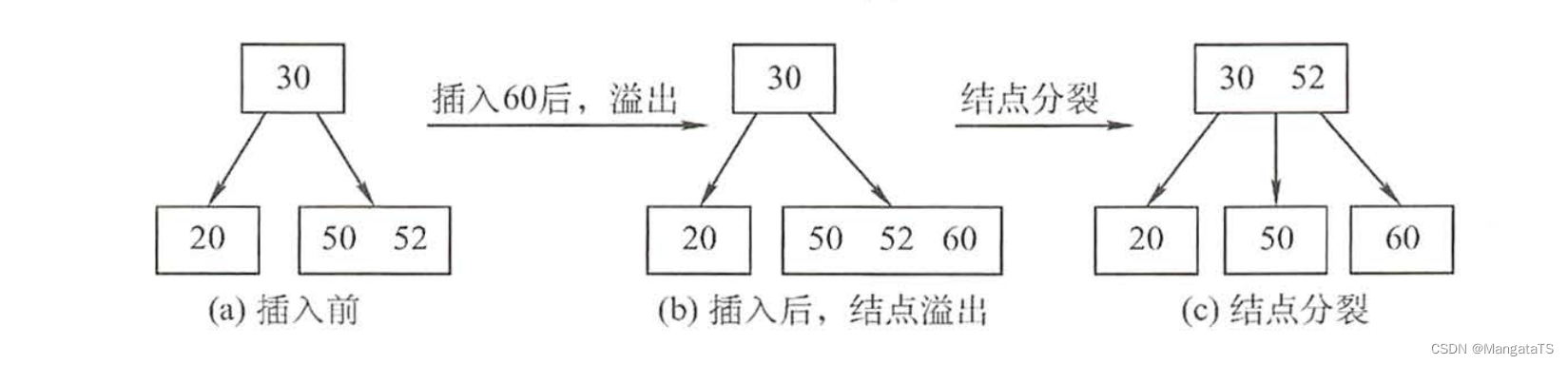我们可以来观察这个过程：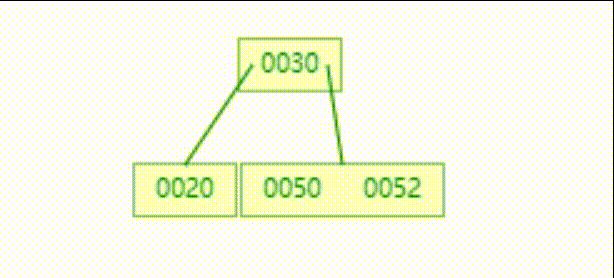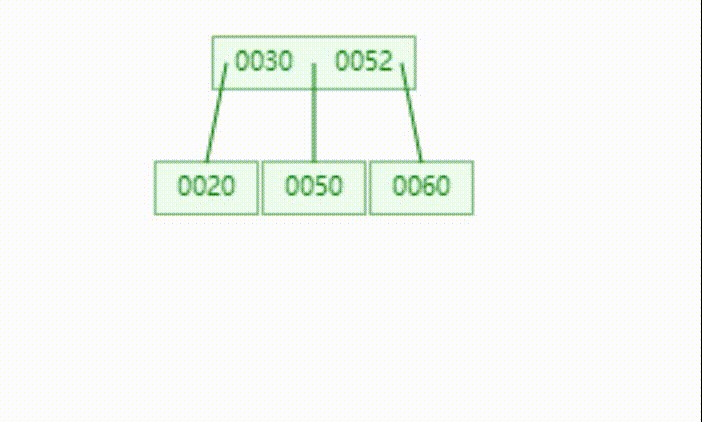### 3.4 删除操作¶

#### 3.4.2 删除关键字是叶子结点¶

• 一、如果删除关键字后该结点的关键字个数仍然大于等于 $$\left \lceil \frac{m}{2} \right \rceil$$ 那么直接删除就完事
• 二、如果删除关键字后该结点的关键字个数等于 $$\left \lceil \frac{m}{2}-1 \right \rceil$$
• ①如果兄弟结点够借，即与此结点同一层的临近的右（左）兄弟结点的关键字个数大于等于 $$\left \lceil \frac{m}{2} \right \rceil$$ 那么就可以将父结点的关键字填充到当前结点，然后再将兄弟结点的关键字移到父结点上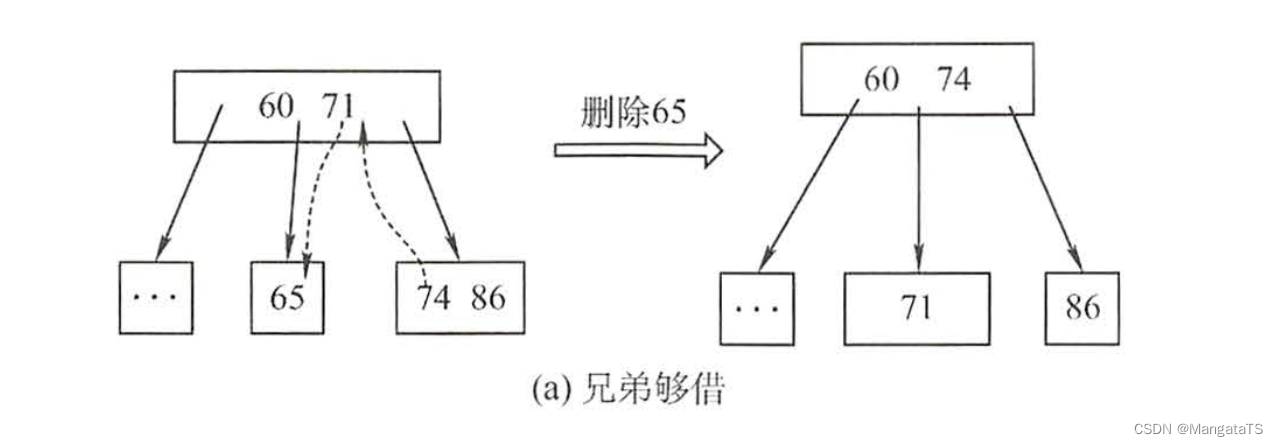• ②如果兄弟结点不够借，即与此结点同一层的临近的右（左）兄弟结点的关键字个数等于 $$\left \lceil \frac{m}{2} -1\right \rceil$$ 那么需要将该结结点删除后，和其左兄弟（或者右兄弟）结点以及父结点进行合并操作，若是合并之后超出了关键字的限制，则进行向上分裂操作

## 四、B+树¶

$$B+$$ 树是从 $$B$$ 树改进过来的，他和 $$B$$ 树的主要区别在于： - $$B+$$ 树中保存元素的结点都在叶子结点上， 非叶子结点只起到索引 的作用 - $$B+$$ 树的所有叶子结点都通过指针链接起来了 - $$B+$$ 树每一个关键字对应一棵子树，假设一个结点最多有 $$n$$ 个关键字，那么其结点只有 $$n$$ 颗子树 - 一个 $$m$$$$B+$$ 树除跟节点外，其余结点的关键字个数 $$n$$ 的范围为 $$\left \lceil \frac{m}{2} \right \rceil<=n<=m$$ ，根节点的关键字区间为：$$1<=n<=m$$

• ①每一个分支节点最多有 $$m$$ 颗子树
• ②非根结点至少有两颗子树，其他每个分支结点至少有 $$\left \lceil \frac{m}{2} \right \rceil$$ 棵子树
• ③结点的子树个数与关键字个数相等
• ④叶结点按照关键字升序（降序）排列，且相邻叶结点通过指针链接起来

## 五、应用¶

B+树主要用于 磁盘IO 的读取， 数据库的索引